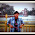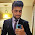## List of All Programs

The Following is the List of all the programs on my Blog

#### Miscellaneous

1.More content needed on blog

2.Sure, Working on it. Thanks for your inputs

3.all contents are very useful.. thanks!! i need playfair cipher using python, can u plz put ur content for that..

4.amazing blog~ It really helps a lot!

### Infix to Prefix conversion using Stack

This post is about conversion of Infix expression to Prefix conversion. For this conversion we take help of stack data structure, we need to push and pop the operators in and out of the stack.

Infix expressions are the expressions that we normally use,eg. 5+6-7; a+b*c etc. Prefix expressions are the expressions in which the 2 operands are preceded by the operator eg. -+567 , +a*bc etc.

This method is very similar to the method that we used to convert Infix to Postfix but the only difference is that here we need to reverse the input string before conversion and then reverse the final output string before displaying it.

NOTE: This changes one thing that is instead of encountering the opening bracket we now first encounter the closing bracket and we make changes accordingly in our code.

So, to convert an infix expression to a prefix expression we follow the below steps
(we have 2 string, 1st is the input infix expression string 2nd is the output string which is empty initially)

We first revers…

Hashing is a technique used for storing , searching and removing elements in almost constant time. Hashing is done with help of a hash function that generates index for a given input, then this index can be used to search the elements, store an element, or remove that element from that index.

A hash function is a function that is used to map the data elements to their position in the data structure used. For example if we use an array to store the integer elements then the hash function will generate position for each element so that searching, storing and removing operation on the array can be done in constant time that is independent of the number of elements in the array. For better look at the example below.

now we face a problem if for 2 numbers same position is generated example consider elements 1 and 14

1 % 13 = 1

14 % 13 = 1

so when we get 1 we store it at the first position, but when we get 14 we see that the position 1 is already taken, this is a case of collision.

Inorder…